# An Introduction to Machine Learning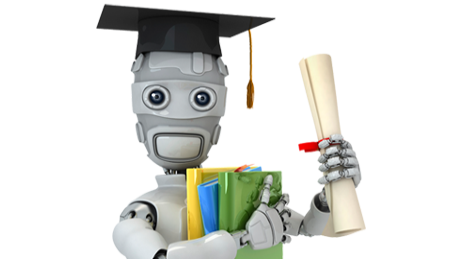Machine learning (a field of artificial intelligence) is a rapidly expanding technology that we see  in use more and more in our daily lives. It is used to give us more accurate results when we do an internet search, suggest products to us when we are shopping, and offer diagnoses to our maladies.

But what exactly is machine learning? How does it work and how is it implemented? There is a lot to cover on this topic, but I want to give you a brief introduction to the main types of machine learning and some of the algorithms used.

Note that most of the math/logic associated with machine learning is available via programming libraries in many languages. That being said, you still need to understand the basic principles of machine learning to know what algorithms and procedures you need to use. Hopefully, this blog will help you out with making the right choices.

## Definition of Machine Learning

To wrap our heads around what machine learning means and what it is trying to do, let’s look at a couple definitions. First, here is the ‘layman’s’ definition:

“(Machine learning is) the field of study that gives computers the ability to learn without being explicitly programmed.” , Arthur Samuel

A more formal definition is:

“A computer program is said to learn from experience E with respect to some class of tasks T and performance measure P, if its performance at tasks in T, as measured by P, improves with experience E.” , Tom Mitchell

So what does that mean? IT is basically saying that a machine is learning if it get’s better at the task is performing the more times it does it. ‘Normal’ algorithms do the same thing every time they are run. Like a fine wine, machine learning algorithms get better with age.

## Types of Machine Learning

There are two main types of machine learning: Supervised and Unsupervised.

### Supervised Learning

In supervised learning, we are given a data set and already know what our correct output should look like. We have the idea that there is a relationship between the input and the output. There are two main types of supervised learning: Regression and Classification.

#### Regression

Regression algorithms are used for results that can be any real number.For example, predicting the price of a house would be a regression problem, since the output can take on any of a wide range of values. One of the most common algorithms used for regression problems is Linear Regression.

#### Classification

Classification means that the result is one of a distinct set of values. Classification includes ‘true/false’ problems such as ‘will this house sell over the asking price?’ It is also used for assigning objects to a particular category. Classification’s go-to algorithm is Logistic Regression (don’t let the regression in the name fool you). Both Linear and Logistic Regression come to us from the world of statistics.

### Unsupervised Learning

Unsupervised learning is a flavor of machine learning in which we do not have a set of data with answers to train on. Unsupervised learning is used to find trends in data. Some examples of how it is used include sorting, market segmentation, anomaly detection, and spam detection.

## The Basics

So how does machine learning work? There is a lot to cover in the field, but for now I just want to introduce some of the basic concepts at a high level. For a supervised learning problem, we can sum up the learning strategy as follows:

• If we have a set of ‘test’ data with a certain number of ‘features’…
• We can form a ‘hypothesis’ function so that…
• When we plug our features into the hypothesis, we can adjust the ‘theta’ parameters for features to minimize the ‘cost’, or error between our predictions and known outcomes

OK, so what does all this mean? Well, features are simply the various data points we are using to help us make our prediction. In our house selling price example, features could be the size of the house in square feet, the number of bedrooms, number of bathrooms, size of the lot, neighborhood average income, etc. Note we can also ‘create’ our own features to help fit our data better. For example, we might use the square of the number of bedrooms (i.e. numBedrooms2) to help our features map to our known outcomes better.

These features are plugged into our hypothesis function. Our hypothesis function looks like this: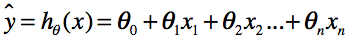Here are a few definitions to help make sense of this:

y = the predicted result
h = our hypothesis function
θ = adjustable parameters of our hypothesis
x = training features for our hypothesis

So we can wiggle our ‘Theta’ (θ) parameter values to make our training features in our hypothesis generate predicted values that map closely to the actual results in our training set. We measure how closely we are matching using the Cost Function. I am not going to bore you with the math, but the Cost Function basically measures the average error across all samples in our training set.

Now that we can measure the cost, we want to minimize it. We want to wiggle our Theta parameters to get the best fit (i.e. least error) for our data. We use ‘minimization functions’ to accomplish this. One common technique is called Gradient Descent. There are many other advanced minimization functions as well. These all serve the purpose of finding the best Theta parameters for our hypothesis; some algorithms are more efficient and can process our data faster.

## Bias & Variance

Bias and Variance are terms used in machine learning that refer to how well your hypothesis is fitting your training data to the actual results. Bias refers to the degree of underfitting of your predicted results to the actual results. Variance refers to the overfitting of your predicted results. Here’s a few graphs to give you a better idea (note we only have 2 features; a lot of concepts in machine learning are explained with 2 features, since we can graph them!):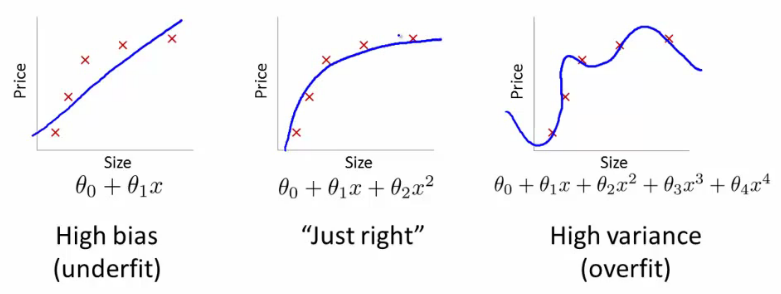How are Bias and Variance used? These metrics give us insight into how we should adjust our hypothesis to get a better fit. We can use Learning Curves to give us insight as to whether our hypothesis is exhibiting high bias or high variance characteristics. In a learning curve, we compare the results from our training set with the results from a cross-validation set.

Typically when training a machine learning algorithm you split your data into a training set (~60% of the samples), corss-validation set (~20% of the samples) and test set (~20% of the samples). Once your machine learning algorithm is tuned with the training and cross-validation sets, the final error can be measured with the test set.

Here are a couple examples of learning curves: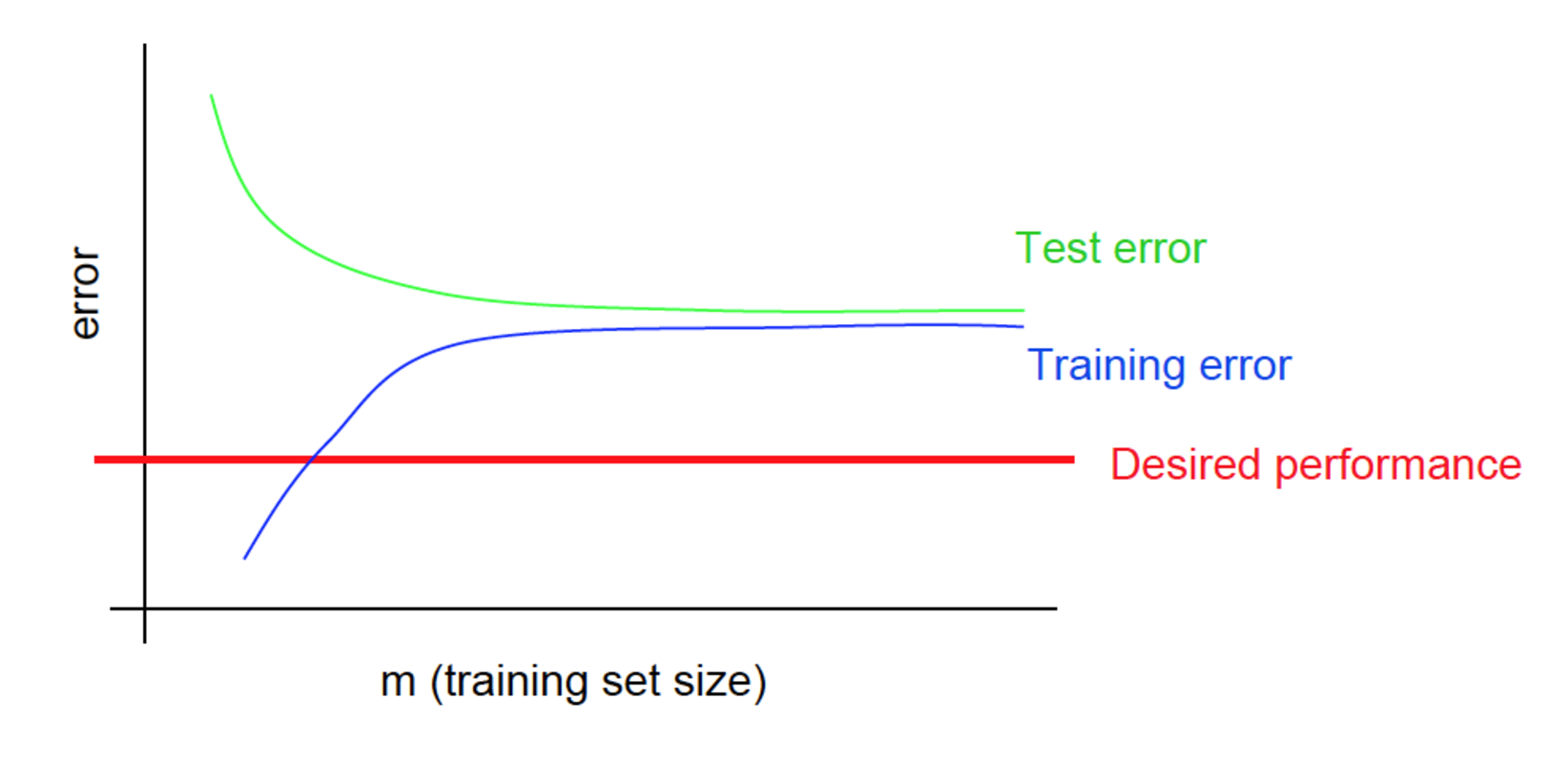A High Bias Learning Curve. Note the training and test error are close together, but higher than the desired performance.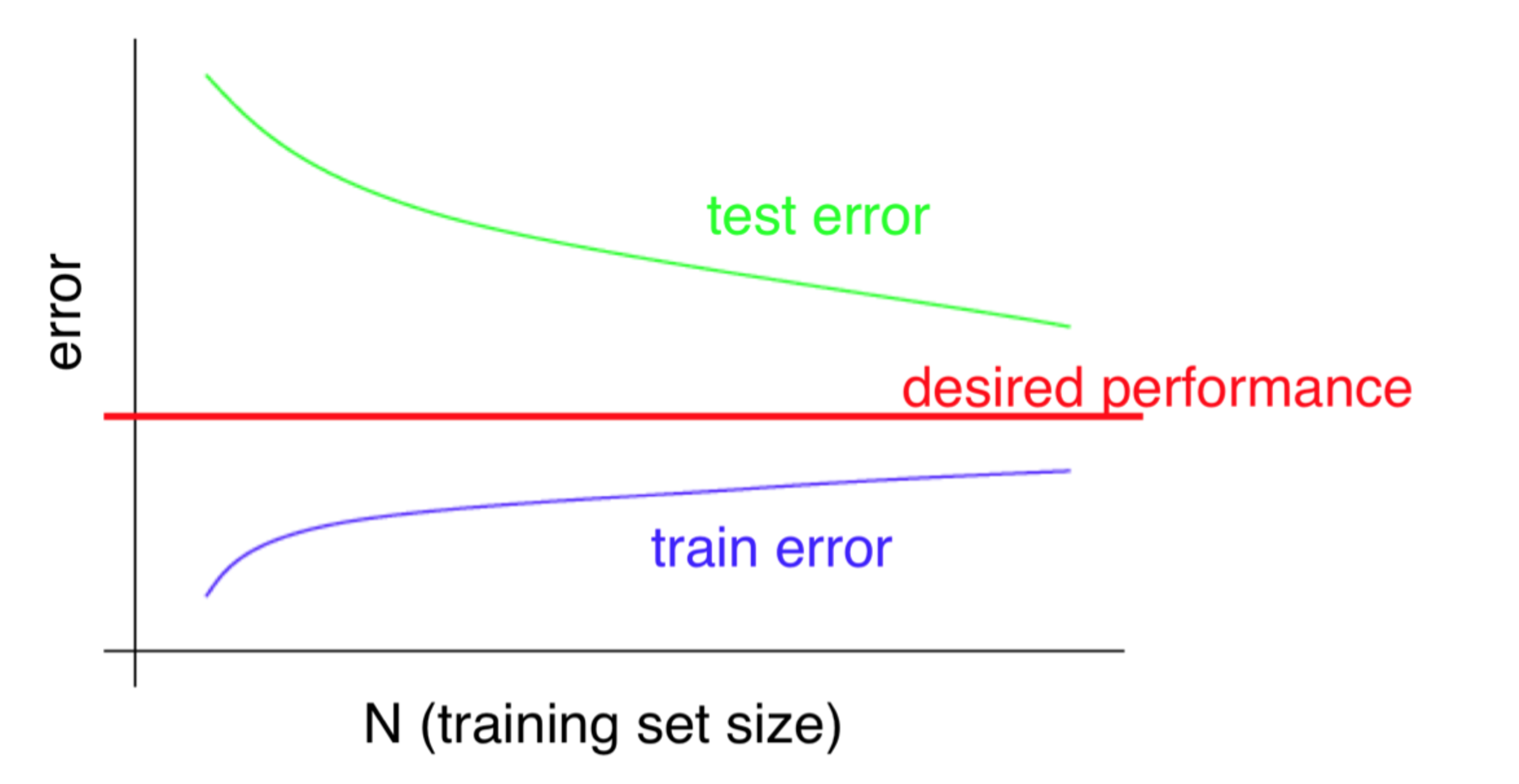A High Variance Learning Curve. Note the training error is better than the desired performance, but the test error is not.

If we have a high bias or high variance problem, what can we do to fix it? We have a few options at hand:

If the problem is high bias, we can try to add more features by either collecting more information per sample, or creating our own polynomial features.

If the problem is high variance, we can reduce the number of features, and/or get more training examples. As you can see in the high variance graph above it looks like test and training error are converging with more samples. This (typically) makes a high variance problem easier to solve than a high bias one. We simply need to gather more data for the algorithm to learn from.

## Error Analysis

Error Analysis is an important aspect of creating a useful machine learning algorithm. This is the process of looking over your results and seeing how you can improve them. Here are some tips about performing error analysis:

• Start with a simple algorithm, implement it quickly, and test it early.
• Plot learning curves to decide if more data, more features, or less features will help.
• Manually examine the errors on examples in the cross validation set and try to spot a trend.
• Get error results as a single, numerical value; otherwise it is difficult to assess your algorithm’s performance.
• You may need to process your input before it is useful. For example, if your input is a set of words, you may want to treat the same word with different forms (fail/failing/failed) as one word, so you could use “stemming software” to recognize them all as one.

## Wrapping Up

We’ve only scratched the surface here regarding some of the basics of machine learning. Hopefully after reading this you have a sense of how machine learning works, what it can be used for and some basics on the implementation of machine learning algorithms.

If you want to learn even more ,I highly recommend this online course by Andrew Ng at Stanford University. It will show you how to implement your own machine learning systems.

You are now primed to dig even deeper into the world of machine learning!